# Multiplying Fractions, Whole Numbers, and Mixed Numbers Lesson Plan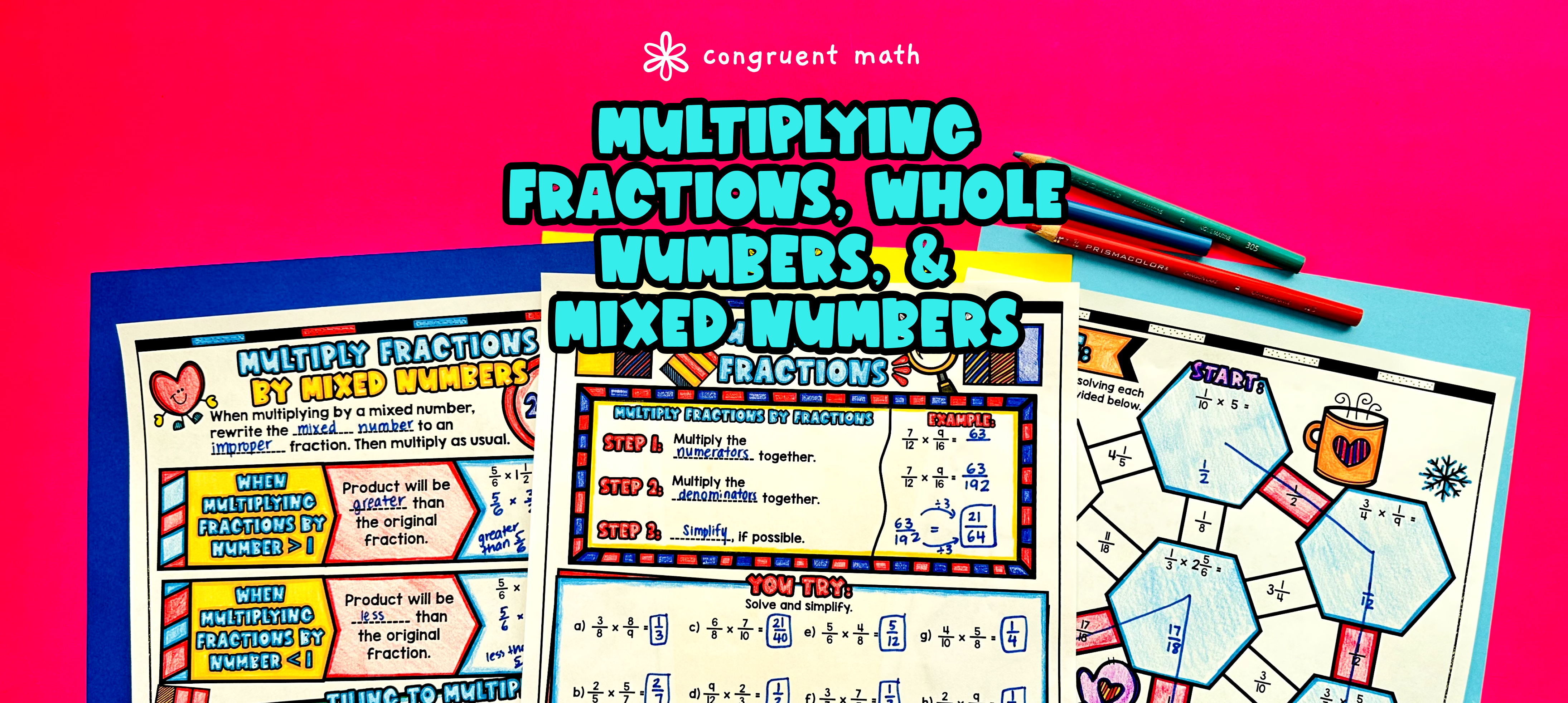Pin This

## Overview

Ever wondered how to teach multiplying fractions, whole numbers, and mixed numbers in an engaging way to your 5th or 6th-grade students?

In this lesson plan, students will learn about fraction multiplication and its real-life applications. Through artistic and interactive guided notes, check for understanding activities, a doodle and color by number worksheet, and a maze worksheet, students will gain a comprehensive understanding of multiplying fractions, whole numbers, and mixed numbers.

The lesson culminates with a real-life example that explores how this math skill can be applied in practical situations. Students will have the opportunity to read and write about real-life uses of multiplying fractions, whole numbers, and mixed numbers, connecting their learning to the world around them.

## Get the Lesson Materials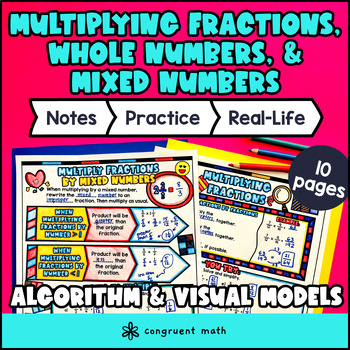\$4.25

## Learning Objectives

After this lesson, students will be able to:

• Multiply fractions by whole numbers using visual models and algorithms

• Multiply fractions by fractions using visual models and algorithms

• Multiply fractions and mixed numbers using visual models and algorithms

• Explain the real life application of fraction multiplication

## Prerequisites

Before this lesson, students should be familiar with:

• Basic multiplication skills of whole numbers

• Understanding of the concept of numerators vs. denominators

• Basic understanding of mixed numbers and improper fractions

## Key Vocabulary

• Multiplying fractions

• Whole numbers

• Mixed numbers

• Visual models

• Algorithm

## Procedure

### Introduction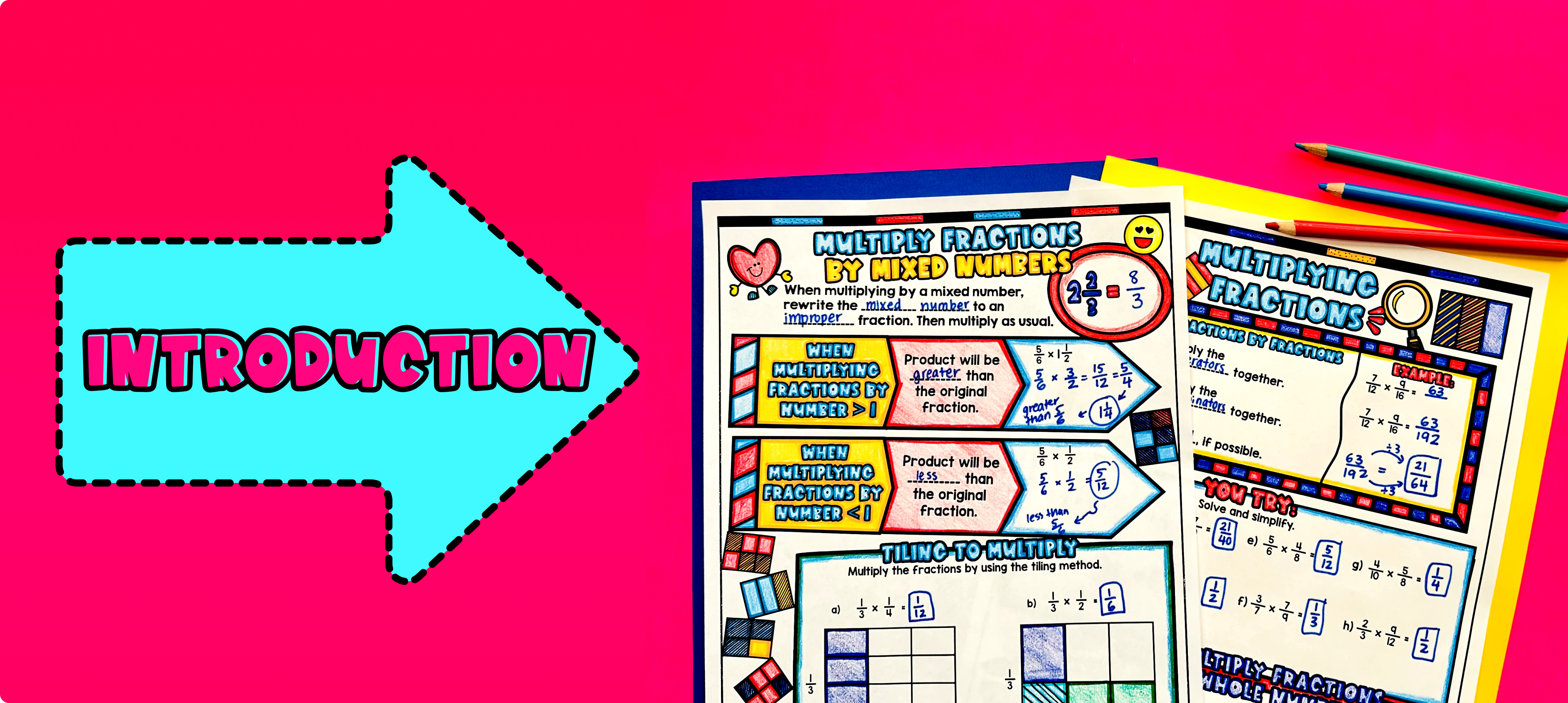Pin This

As a hook, ask students why multiplying fractions, whole numbers, and mixed numbers is an important skill in real life. Refer to the real-life application page included in this resource for ideas. You can also ask questions like "Have you ever had to multiply a fraction and a whole number?" or "Can you think of a situation where you would need to multiply mixed numbers?" This will help activate their prior knowledge and engage them in the lesson.

Use the first page of the guided notes to introduce the concept of multiplying fractions by whole numbers. Walk through the key points of this topic, including how to set up the multiplication problem, multiply the numerator and the whole number, and simplify the fraction if necessary. Also, take some time to go through how to multiply fractions by whole numbers (bottom of page 1 of guided notes). Emphasize the visual models provided in the guided notes to help students understand the concept.

Introduce how to multiply fractions by mixed numbers using the second page of the guided notes. Introduce visual diagrams and tiling (bottom of page 2 of guided notes).

Based on student responses, reteach concepts that students need extra help with. If your class has a wide range of proficiency levels, you can pull out students for reteaching, and have more advanced students begin work on the practice exercises.

### Practice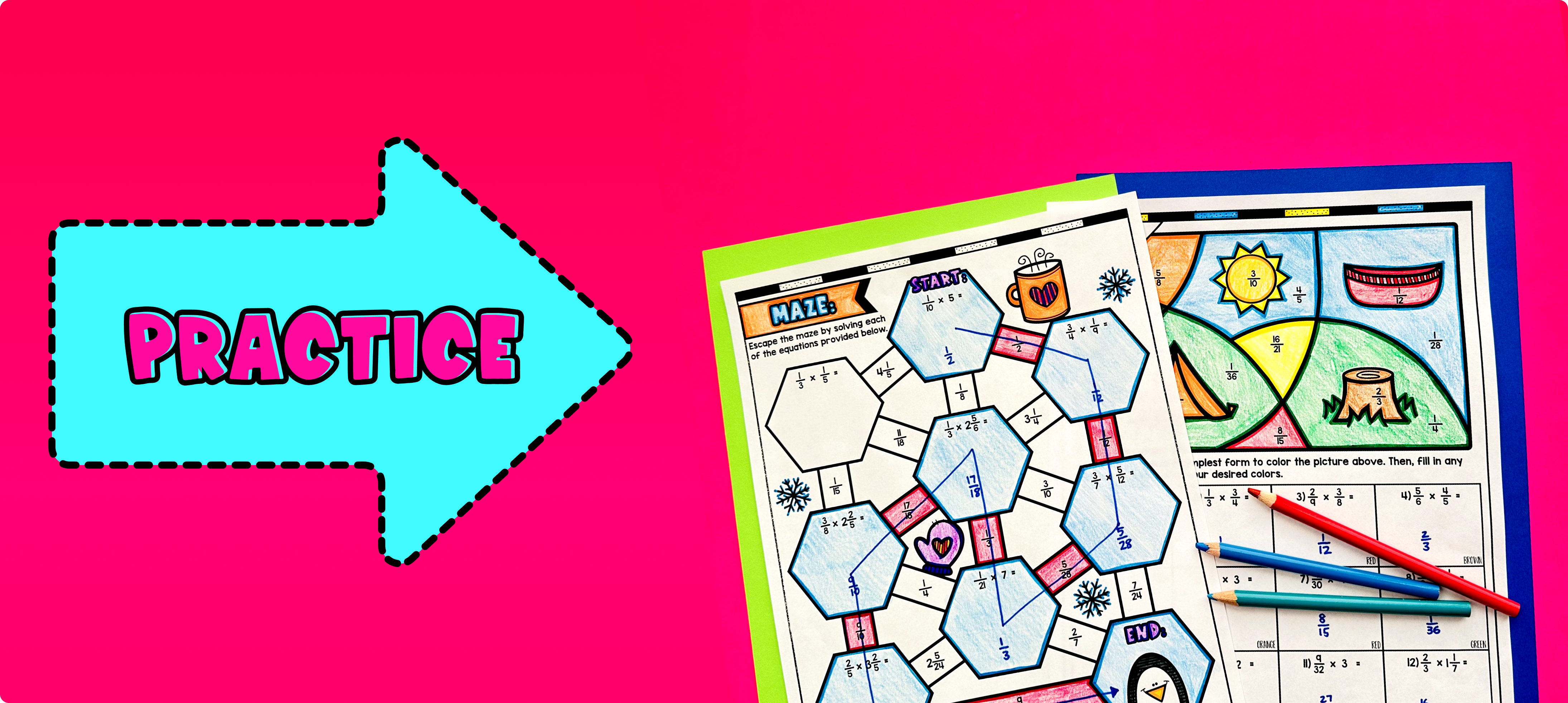Pin This

Have students practice multiplying fractions, whole numbers, and mixed numbers using the maze activity (page 3 of guided notes). Walk around to answer student questions.

Fast finishers can dive into the color by number activity for extra practice (page 4 of guided notes). You can assign it as homework for the remainder of the class.

### Real-Life Application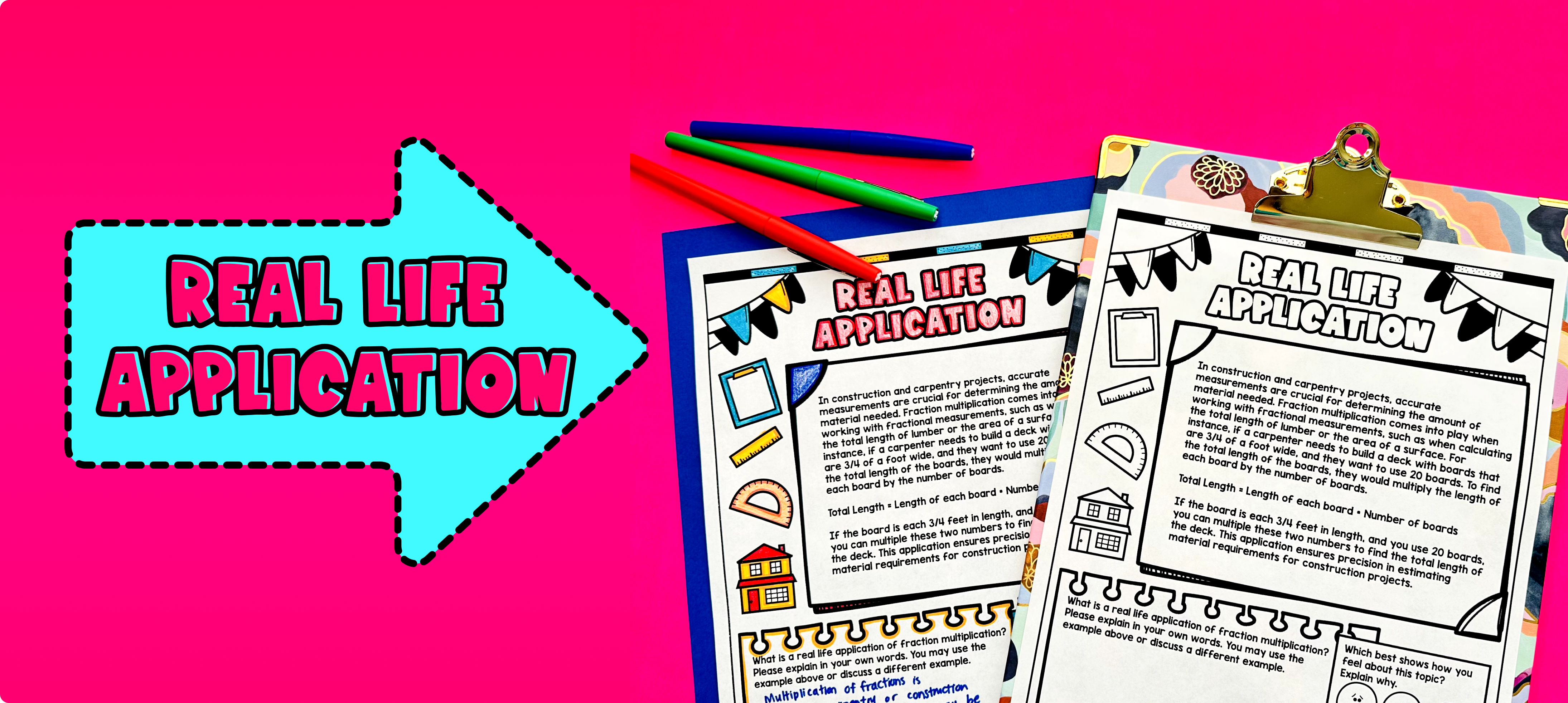Pin This

Use the last page of the guided notes to bring the class back together, and introduce the concept of real-life applications of multiplying fractions, whole numbers, and mixed numbers. Explain to the students that understanding how to multiply these types of numbers can be useful in various real-life situations.

For example, when baking or cooking, we often need to adjust a recipe to make more or less food. Sometimes, the recipe is written for a certain number of servings, and we need to double or halve the ingredients. By knowing how to multiply fractions, whole numbers, and mixed numbers, we can easily scale the recipe to fit our needs.

Another example is when building or constructing. Architects, engineers, and carpenters often need to calculate measurements and dimensions when designing and building structures. By knowing how to multiply fractions and whole numbers, they can accurately determine the amount of materials needed.

Refer to the FAQ section for more ideas on how to teach the real-life application of multiplying fractions, whole numbers, and mixed numbers.